### Home > CALC > Chapter 7 > Lesson 7.3.5 > Problem7-157

7-157.
1. Integrate. Show your steps—if you use u-substitution be sure to change the bounds of integration. Homework Help ✎

1.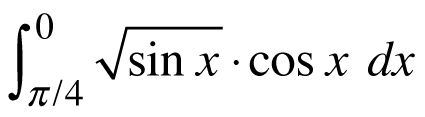2.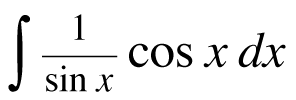3.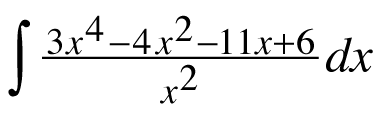4.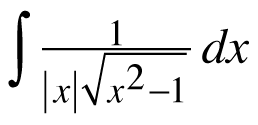5.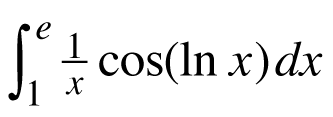6.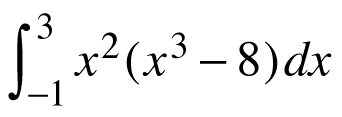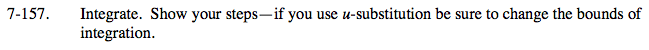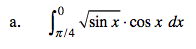U = sinx
dU = cosx dx

$U\left ( \frac{\pi }{4} \right )=\text{ lower bound}$

U(0) = upper bound

Assemble the integral and then solve.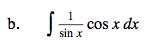U = sinx
dU = cosx dx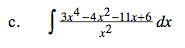Before you integrate, rewrite the integrand.

$= \int 3x^{2}-4-\frac{11}{x}+\frac{6}{x^{2}}dx=$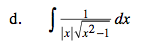sec−1x + C (this is a special case)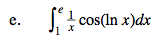$\frac{d}{dx}\left ( \frac{1}{x} \right )=\text{ln}x$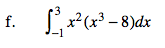You could use U-Substitution.
Or you could expand the integrand and evaluate.